Courses

# Quant Mock Test - 3

## 15 Questions MCQ Test Mock Test Series for CLAT 2020 | Quant Mock Test - 3

Description
This mock test of Quant Mock Test - 3 for CLAT helps you for every CLAT entrance exam. This contains 15 Multiple Choice Questions for CLAT Quant Mock Test - 3 (mcq) to study with solutions a complete question bank. The solved questions answers in this Quant Mock Test - 3 quiz give you a good mix of easy questions and tough questions. CLAT students definitely take this Quant Mock Test - 3 exercise for a better result in the exam. You can find other Quant Mock Test - 3 extra questions, long questions & short questions for CLAT on EduRev as well by searching above.
QUESTION: 1

### Directions: Study the following graph carefully to answer the questions –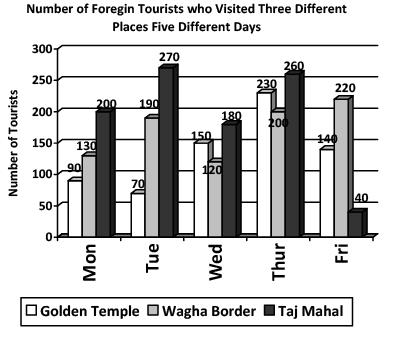Q. What is the average number of foreign tourists who visited Wagha Border on all the days together?

Solution: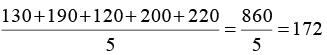QUESTION: 2

Solution:
QUESTION: 3

### Directions: Study the following graph carefully to answer the questions –Q. What is the respective ratio between the number of foreign tourists who visited Taj Mahal on Thursday and the number of foreign tourists who visited Golden Temple on Friday?

Solution:
QUESTION: 4

Directions: Study the following graph carefully to answer the questions –Q. On which day was the total number of foreign tourists who visited all the three places together second lowest?

Solution:
QUESTION: 5

Directions: Study the following graph carefully to answer the questions –Q. Total number of foreign tourists who visited all the three places on Tuesday together is what per cent of the total number of foreign tourists who visited Wagha Border on Monday and Wednesday together?

Solution:

The correct option is D.

Explanation-

Total no. of foreign tourists who visited all the three places on tuesday = 70+190+270 = 530

Total no. of foreign tourists who visited wagha border on monday and wednesday = 130+120 = 250

Percentage of  the total number of foreign tourists who visited Wagha Border on Monday and Wednesday together = 530/250*100 = 212.

QUESTION: 6

Directions: Study the following piechart carefully and answer the questions given below:
Preferences of students for six chocolates in terms of degrees of angle in the pie-chart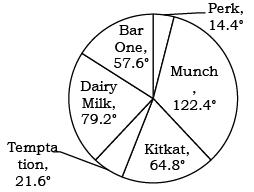Total number of students = 6800

Q. What is the difference between the total number of students who prefer Dairy Milk and Perk together and the total number of students who prefer Munch and Temptation together?

Solution: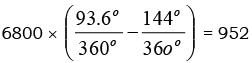QUESTION: 7

Directions: Study the following piechart carefully and answer the questions given below:
Preferences of students for six chocolates in terms of degrees of angle in the pie-chartTotal number of students = 6800

Q. What is the ratio of the number of students who prefer Temptation to the number of students who prefer Dairy Milk?

Solution:

21.6° : 79.2
3 : 11

QUESTION: 8

Directions: Study the following piechart carefully and answer the questions given below:
Preferences of students for six chocolates in terms of degrees of angle in the pie-chartTotal number of students = 6800

Q. The number of students who prefer KitKat and Temptation together is what per cent of the total number of students?

Solution: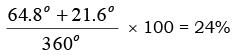QUESTION: 9

Directions: Study the following piechart carefully and answer the questions given below:
Preferences of students for six chocolates in terms of degrees of angle in the pie-chartTotal number of students = 6800

Q. The number of students who prefer Perk is approximately what per cent of the number of students who prefer Munch?

Solution: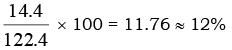QUESTION: 10

Directions: Study the following piechart carefully and answer the questions given below:
Preferences of students for six chocolates in terms of degrees of angle in the pie-chartTotal number of students = 6800

Q. What is the ratio of the average of the students who prefer Dairy Milk, KitKat and Temptation together to those who prefer BarOne, Perk and Munch together?

Solution: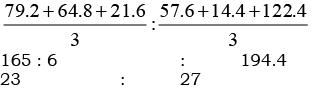QUESTION: 11

Directions: Study the following table carefully. Some values are missing. Complete that based on given information in each question to answer the question.
The table shows the number of employees in an organization in 5 different cities with total employees being 2130 in the organization. Table also shows the percentage of employees working in 4 departments – HR, Finance, Software and Accounts with each employee in only 1 department.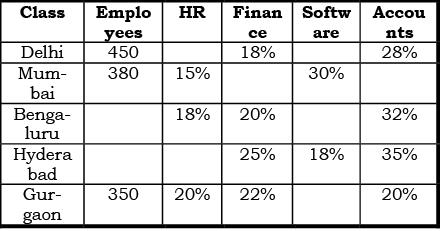Q. What is the total number of employees in Mumbai and Gurgaon who work in Software department?

Solution:

In Gurgaon, Software % = 100 – (20 + 22 + 20) = 38%
Required Ans = (30/100) * 380 + (38/100)*350

QUESTION: 12

Directions: Study the following table carefully. Some values are missing. Complete that based on given information in each question to answer the question.
The table shows the number of employees in an organization in 5 different cities with total employees being 2130 in the organization. Table also shows the percentage of employees working in 4 departments – HR, Finance, Software and Accounts with each employee in only 1 department.Q. If in Delhi, employees who work in Accounts department are 40% more than employees who work in HR department, then what is the number of employees who work in Software department in Delhi?

Solution:

Let % of employees who work in HR in Delhi is x%.
So [28/100 * 450 – x/100 * 450]/[x/100 * 450] * 100 = 40
Which is [(28 - x)/x] * 100 = 40
Solve, x = 20
So % of employees who work in Software is 100 – (20 + 18 + 28) = 34%
So required ans = 34/100 * 450

QUESTION: 13

Directions: Study the following table carefully. Some values are missing. Complete that based on given information in each question to answer the question.
The table shows the number of employees in an organization in 5 different cities with total employees being 2130 in the organization. Table also shows the percentage of employees working in 4 departments – HR, Finance, Software and Accounts with each employee in only 1 department.Q. If number of employees in Bengaluru is 10% less than number of employees in Hyderabad, then what is the difference between number of employees who work in Accounts department in these 2 cities and who work in Finance department in these 2 cities?

Solution:

Total employees in Bengaluru and Hyderabad = 2130 – (450 + 380 + 350) = 950
So if in Hyderabad, employees is x, then in Bengaluru – 90/100 * x So x + 90x/100 = 950
Solve, x = 500,
so in Hyderabad = 500, and in Bengaluru = 90/100 * 500 = 450
So required answer = [32/100 * 450 + 35/100 * 500] – [20/100 * 450 + 25/100 * 500]
Or = 12/100 * 450 + 10/100 * 500

QUESTION: 14

Directions: Study the following table carefully. Some values are missing. Complete that based on given information in each question to answer the question.
The table shows the number of employees in an organization in 5 different cities with total employees being 2130 in the organization. Table also shows the percentage of employees working in 4 departments – HR, Finance, Software and Accounts with each employee in only 1 department.Q. If a same criterion as taken in question 33 is taken, in which city the total employees who work in Finance and Accounts departments is more?

Solution:

Delhi – (18 + 28)/100 * 450 = 207
Mumbai– 55/100 * 380 = 209
Bengaluru – (20 + 32)/100 * 450 = 234
Hyderabad – (25 + 35)/100 * 500 = 300
Gurgaon – (22 + 20)/100 * 350 = 147

QUESTION: 15

Directions: Study the following table carefully. Some values are missing. Complete that based on given information in each question to answer the question.
The table shows the number of employees in an organization in 5 different cities with total employees being 2130 in the organization. Table also shows the percentage of employees working in 4 departments – HR, Finance, Software and Accounts with each employee in only 1 department.Q. If in Mumbai, number of employees who work in Accounts department is 19 more than the number of employees who work in Software department in Gurgaon, then what is the number of employees who work in Finance department in Mumbai?

Solution:

% of employees who work in Software in Gurgaon = 100 – (20 + 22 + 20) = 38%
Number of employees who work in Software in Gurgaon = 38/100 * 350 = 133
So number of employees who work in Accounts in Mumbai is 133 + 19 = 152
So number of employees who work in Finance in Mumbai = 380 – [152 + ((15 + 30)/100 * 380)] = 57